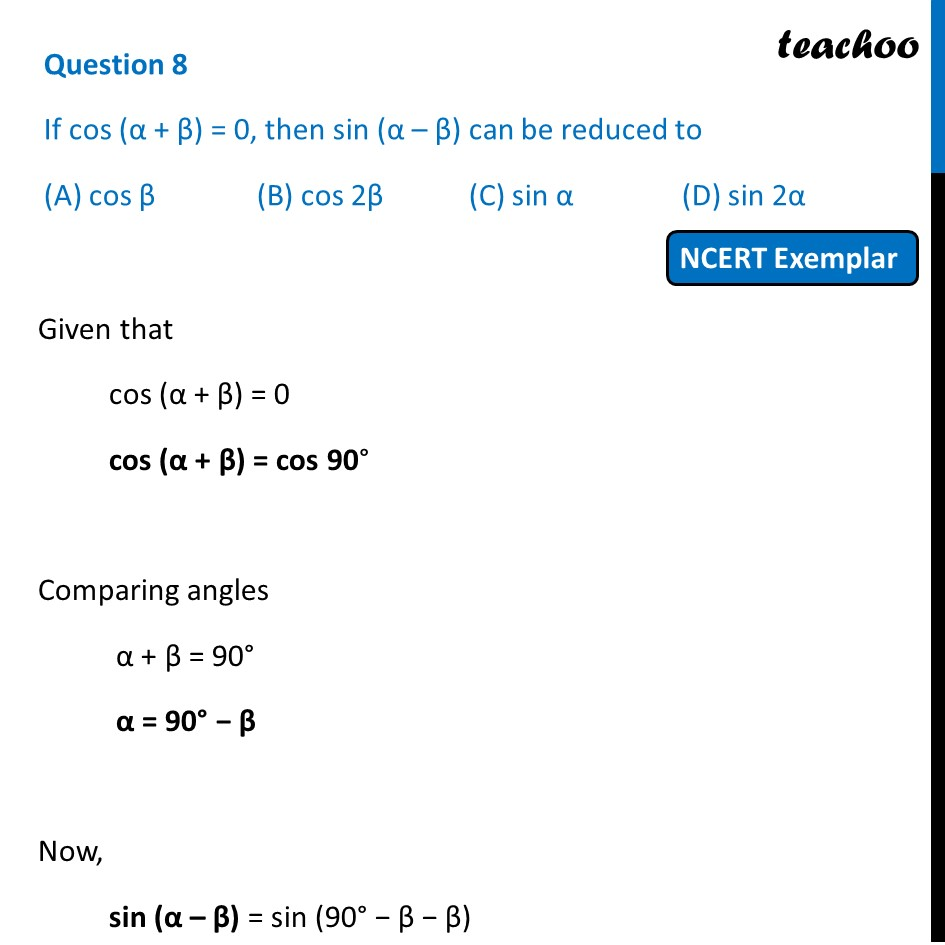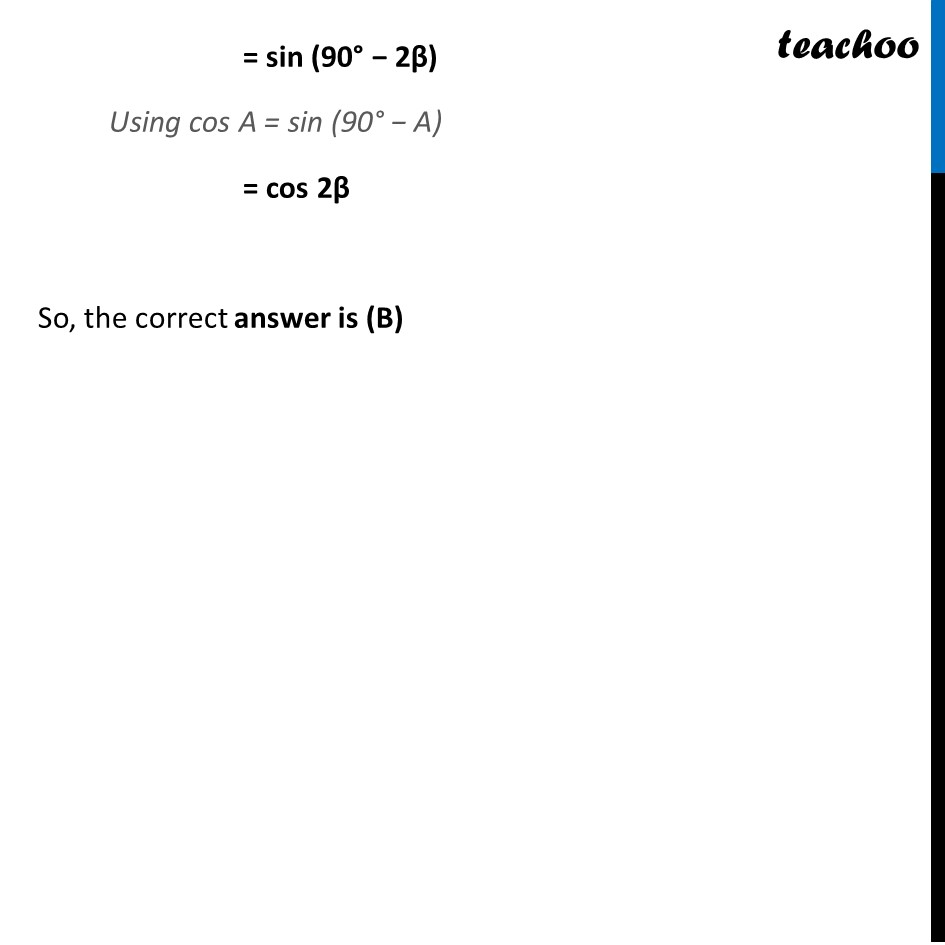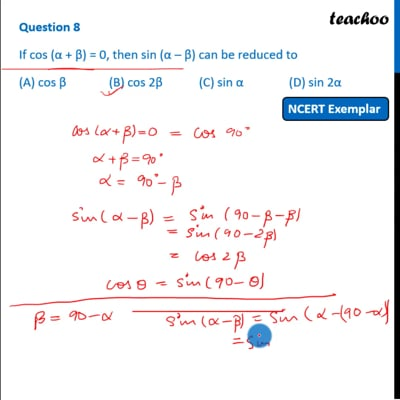NCERT Exemplar - MCQ

Chapter 8 Class 10 Introduction to Trignometry (Term 1)
Serial order wise

## (A) cos β   (B) cos 2β   (C) sin α   (D) sin 2αThis video is only available for Teachoo black users

### Transcript

Question 8 If cos (α + β) = 0, then sin (α – β) can be reduced to (A) cos β (B) cos 2β (C) sin α (D) sin 2α Given that cos (α + β) = 0 cos (α + β) = cos 90° Comparing angles α + β = 90° α = 90° − β Now, sin (α – β) = sin (90° − β − β) = sin (90° − 2β) Using cos A = sin (90° − A) = cos 2β So, the correct answer is (B)